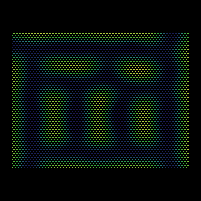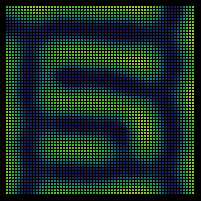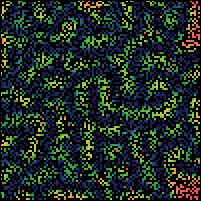# Gray Scott Model of Reaction Diffusion

### Abelson, Adams, Coore, Hanson, Nagpal, Sussman

Reaction and diffusion of chemical species can produce a variety of patterns, reminiscent of those often seen in nature. The Gray Scott equations model such a reaction. For more information on this chemical system see the articles "Complex Patterns in a Simple System," by John E. Pearson and "Pattern Formation by Interacting Chemical Fronts," by K.J. Lee, W.D. McCormick, Qi Ouyang, and H.L. Swinney. These articles appeared in Science, Volume 261, 9 July 1993.U and V and P are chemical species. u and v represent their concentrations. ru and rv are their diffusion rates. k represents the rate of conversion of V to P. f represents the rate of the process that feeds U and drains U,V and P. The partial differential equations modeling this process may be simulated with a variety of numerical techniques. One may expect good results with even such crude methods as forward Euler integration of the finite-difference equations that one obtains by spatial discretization of the Laplacian. One may also simulate the underlying process, which is stoichiometrically conservative (accounting for the source of U and the drain of U, V, and P.) The fact that all the interactions are local makes this a good candidate for a parallel implementation. Diffusion may be modeled by an explicitly conservative exchange process among neighbors where the reactions are locally modeled at each locus by a simulated processor.

Xmorphia shows a beautiful presentation of a simulation of the Gray-Scott reaction diffusion mechanism using a uniform-grid finite-difference model running on an Intel Paragon supercomputer. We are interested in being able to make such simulations with an amorphous computer where the precise positions of the individual processing elements is uncertain.

The physical world appears to be homogeneous and isotropic at the scale of atoms and molecules, so the precise positions of the reaction sites should not be essential to the character of the patterns produced by the chemistry. We can test this by comparing a simulation of similar situations using a crystalline array or an amorphous computer. The simulator presented here allows us to conveniently make this comparison.

You can run a Java version of our simulator to see for yourself how the patterns are formed

### Gray Scott Simulator Demo

The following figures, made with a simulator written in Scheme, illustrate the kind of patterns that can be obtained by varying the grid. In these simulations each locus of reaction is represented by a simulated processor. The processors are asychronous: each one runs its simulation steps at randomly chosen times. Each simulation step is stoichiometrically conservative. In each of these figures we have 4000 loci of reaction. The parameters are: f = 0.04, k = 0.06, r_u = 0.01, r_v = 0.005. The neighborhood radius is 0.04. (So each simulated processor has approximately 20 neighbors.)
The colors show the concentration of U at each locus. Red labels the maximum concentration and Blue labels the minimum concentration. The patterns that you see are stable -- no significant change will occur, regardless of how long we run the process. Each pattern starts off from the same slightly randomized initial condition. The differences among the patterns is due to either the differences of the grids or the differences in the initial condition.Amorphous layout (random, smoothed)Hexagonal close packed layoutSquare layoutAnother Amorphous layout

Although the details differ, we can see that the general character of the patterns developed are similar, independent of the grid. The features have similar sizes and shapes. They are somewhat aligned with the boundaries.

If we decrease the radius of the neighborhood to 0.03, thereby decreasing the average number of neighbors to about 11 (4000*3.14*0.03*0.03), we get correspondingly more delicate features.Amorphous layout (random, smoothed)Hexagonal close packed layout
Interestingly, as the amorphous layout shows, the alignment of the features with the boundary is less sure as the features become small by comparison to the figure.

If you have a fast network connection, you can view some MPEG movies we've made with the asychronous Scheme simulator that show how the process evolves. The first three movies show evolution with the same set of parameters, f = 0.025, k = 0.06, r_u = 0.01, r_v = 0.005. In each movie the reaction occurs at 4000 sites with a neighborhood radius of 0.04. The entire image is of unit size in both the horizontal and vertical direction. In these movies the reaction is occuring asynchronously in that at each instant a random site is activated to do one conservative reaction step. We consider 4000 such individual reaction steps (one for each locus) to be "equivalent" to one full step of a synchronous simulation. Each movie spans 6400 full steps. The movie is made by making frames that periodically sample at integral numbers of full steps.

What is important to notice here is how the qualitative nature of the evolution is independent of the layout of the loci of reaction, and also that the use of asynchronous update produces similar results to those produced by the synchronous simulation performed by the Java simulator we provide, and by the traditional finite-difference results of Xmorphia.

The first movie shows the evolution on an amorphous layout. This movie's frames are taken at 16-step intervals.

The second movie shows the same parameters running on a hexagonal grid. This movie's frames are taken at 16-step intervals.

The third movie shows them running on a square grid, but this time each frame is taken at 8-step intervals, so the picture evolves more slowly.

Our fourth movie shows a different set of parameters, f = 0.024, k = 0.055, r_u = 0.01, r_v = 0.005. Like the first movie, this is running on an amorphous layout of 4000 loci, neighborhood radius 0.04, 16 steps per frame.

The following movies were made using the Java synchronous simulator . Like the asynchronous Scheme simulations above, there were 4000 loci, the neighborhood radius was 0.04, and the processors were placed randomly in the unit square. Frames in each movie were taken at 10 step intervals, and each simulation was allowed to run for at least 5400 steps. The diffusion parameters and the time step were kept constant at r_u = 0.01, r_v = 0.005 and dt = 1.0 for each simulation.

1. #### f = 0.04, k = 0.06 (5,787,741 Bytes)

This simulation provides a basis for comparison between the two simulators. Notice that the stationary patterns formed by the two simulators are qualitatively similar even though the Java simulator updates synchronously while the other does not.

2. #### f = 0.035, k = 0.065 (4,642,042 Bytes)

The pattern formed in this simulation is reminiscent of a leopard's skin. The manner in which the "spots" form is interesting in itself because the process closely resembles cell division.

The following two simulations demonstrate dynamic patterns. That is, no stationary pattern forms within any reasonable amount of time (simulation 4 was run for over 800,000 steps, and there was no visible sign of any less activity than there was at say 4000 steps). These were included to show that the amorphous simulations can qualitatively capture both the static and dynamic patterns described in Pearson's article.

3. #### f = 0.012, k = 0.05 (6,119,376 Bytes)

This pattern is probably best described as "wandering bubbles." The bubbles repeatedly collide with and annihilate each other, only to be regenerated from the residual patterns of those collisions.

4. #### f = 0.025, k = 0.05 (5,297,572 Bytes)

This pattern is characterized by colliding "waves" of color. Large contiguous sections of the field will assume a single color, only to be quickly displaced by the insurgence of another color, that usually originates from an edge or corner of the field.

Belorussian translation